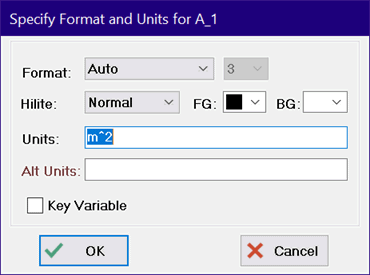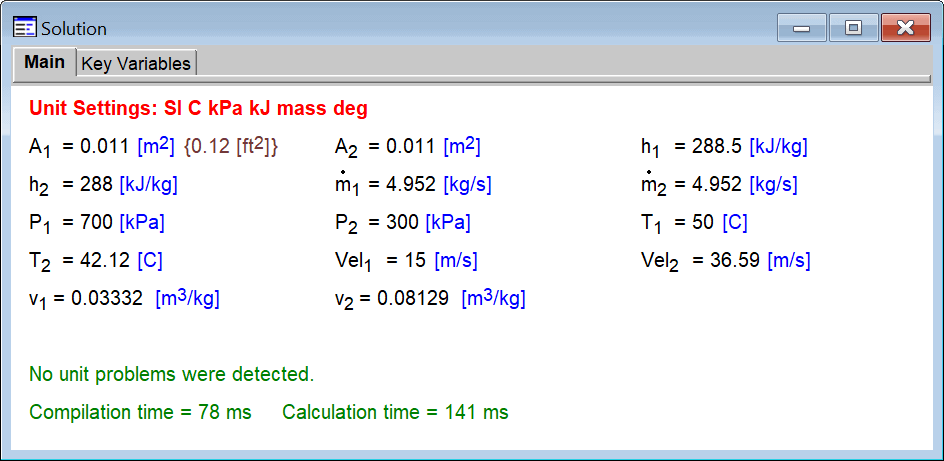Contents - Index

Entering Units

It is necessary to enter the units of each variable for the checking process to function.  There are five ways to enter the units for variables as indicated below.  Regardless of how the units are entered, there must be a separator between each unit designation.  The separator can be a space ( ), hyphen (-), a division sign (/) or a dot (·) which is entered from an extended keyboard as Alt-250 .

All units appearing to the right of a division sign are assumed to be in the denominator unless the star (*) symbol or parentheses are used.  For example, the unit designation, W/m^2-K indicates that the m^2 and K are in the denominator.  A hyphen, space or dot separate units in the numerator or denominator.  Thus W/m^2-K is exactly the same as W/m^2·K  or W/m^2 K or W m^-2 K^-1 and the unit specification could be entered in any of these ways.

The star operator (*), when used after a symbol in the denominator, will place the unit designation in the numerator, unless parentheses are applied.  Therefore W/m^2*K produces the same units as W-K/m^2.  However W/(m^2*K) will result in W/m^2-K.

Dimensionless variables can be designated with a empty unit designation or with a hyphen (-).

1.  Entering units in the Equations Window

The units of a numerical constant in the Equations window can be set by enclosing the units in square brackets following the value.  For example:

P=140 [kPa]

When EES executes this statement, it will recognize that P is set to a numerical constant and the units of P will set to those of the constant, unless they are already set to something else.  A comment can be embedded within a unit string by surrounding the comment with {­ } or  \ characters, e.g., h=100 [kJ/kg\CH4\]  The comment will be displayed with the unit but it will be ignored by the Convert function when doing unit conversions. If the units of variable are entered in the Equations window, the comment within the  {­ } will be removed during compilation and it will not display in the Solution window or elsewhere.  Comments within the backslash characters will be displayed.

Note: The units of any variable appearing in the Equations window can be most easily set by clicking on the variable in the Variable Information Palette.  Click the header cell of the palette if it needs to be updated with recent changes in the Equations window.

Note that the units and alternate units can be selected from a list of units by right-clicking while the cursor is positioned in Equations window.  If an alternate set of units is provided, it must have the same dimensions as the primary set.  In this case, the variable will be displayed in the solution window in both sets of units, as shown.Variables that are specified to be in Time Format are internally assumed to have units of hours since the time is internally converted to a decimal value representing hours.

2.  Entering units in the Solution Window

After solving an equation set, variables are displayed in the Solution window.  The units (and alternative units) of a variable can be entered from the Solution window by clicking the right mouse button on the variable.  The units of more than one variable can be set at one time by clicking the left mouse button on each variable that is to have its units changed and then clicking the right mouse button.  The units of all selected variables will be changed.

3.  Entering units in the Variable Information dialog window

Variables in the main program and in modules and subprograms can be entered in the Variable Information dialog window.  A similar window is provided for internal functions and procedures when right-clicking on an equation in the Debug window while using the Check Units command.  A column is provided to enter the units of the variable.  An alternative set of units (which should have the same dimensions as the primary set) can be entered by selecting (Units) from the drop-down box at the top of the column.

4.  Entering units for Variables in the Parametric or Arrays tables

The units (and alternate units) of variable that appear in the Parametric or Arrays tables can be set by right-clicking in the column header cell and selecting Properties from the pop-up menu.  This action will bring up the Format Table dialog that allows the units and other specifications to be made for the variable represented by the column.

5.  Entering units in the Variable Information palette

A palette listing all variables used in the equations is displayed on the right side of the Equations window.  Clicking on a variable name in the palette will display an abbreviated form of the Variable Information dialog in which the units and other information pertaining to the selected variable can be viewed or changed.

The checking algorithm cannot know the units of pure constants that do not have dimensional information, so it is best to avoid them in your equations.  Instead it would be preferable to set the units of the constants (by appending the units in brackets), define constants or use the Convert function.  For example, suppose you have two variables, L_inch and L_feet, whose units are set to inches and feet, respectively which are used in the following equation.

L_inch=L_feet*12

When the Check Units command is issued, the Debug window will appear and this equation will be flagged as having an error because the units of 12 are not known.

There are several ways to correct this problem.  Perhaps the simplest is to assign units to the numerical constant, 12, so that the equation reads

L_inch=L_feet*12 [in/ft]

Alternatively, the Convert function can be employed as shown next

L_inch=L_feet*convert(ft,in)

See also: Solution Window